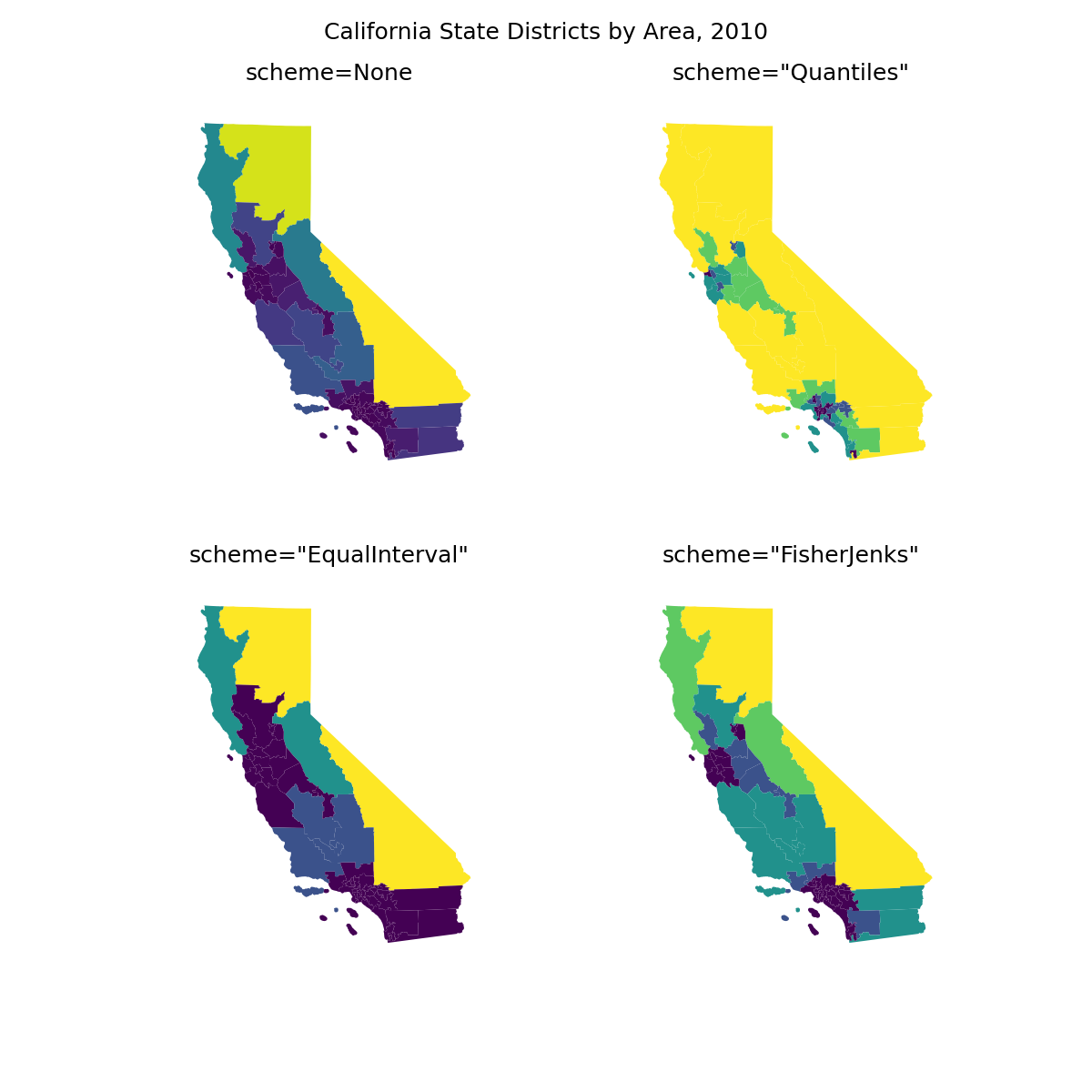# Choropleth of California districts with alternative binning schemes¶

This example demonstrates the continuous and categorical binning schemes available in `geoplot` on a sample dataset of California congressional districts. A binning scheme (or classifier) is a methodology for splitting a sequence of observations into some number of bins (classes). It is also possible to have no binning scheme, in which case the data is passed through to `cmap` as-is.

The options demonstrated are:

• scheme=None—A continuous colormap.

• scheme=”Quantiles”—Bins the data such that the bins contain equal numbers of samples.

• scheme=”EqualInterval”—Bins the data such that bins are of equal length.

• scheme=”FisherJenks”—Bins the data using the Fisher natural breaks optimization procedure.

This demo showcases a small subset of the classifiers available in `mapclassify`, the library that `geoplot` relies on for this feature. To learn more about `mapclassify`, including how you can build your own custom `UserDefined` classifier, refer to the mapclassify docs.Out:

```/Users/alekseybilogur/Desktop/geoplot/examples/plot_california_districts.py:35: UserWarning: Geometry is in a geographic CRS. Results from 'area' are likely incorrect. Use 'GeoSeries.to_crs()' to re-project geometries to a projected CRS before this operation.

cali = cali.assign(area=cali.geometry.area)
/Users/alekseybilogur/Desktop/geoplot/examples/plot_california_districts.py:46: UserWarning: Geometry is in a geographic CRS. Results from 'area' are likely incorrect. Use 'GeoSeries.to_crs()' to re-project geometries to a projected CRS before this operation.

scheme = mc.Quantiles(cali.area, k=5)
/Users/alekseybilogur/Desktop/geoplot/examples/plot_california_districts.py:52: UserWarning: Geometry is in a geographic CRS. Results from 'area' are likely incorrect. Use 'GeoSeries.to_crs()' to re-project geometries to a projected CRS before this operation.

scheme = mc.EqualInterval(cali.area, k=5)
/Users/alekseybilogur/Desktop/geoplot/examples/plot_california_districts.py:58: UserWarning: Geometry is in a geographic CRS. Results from 'area' are likely incorrect. Use 'GeoSeries.to_crs()' to re-project geometries to a projected CRS before this operation.

scheme = mc.FisherJenks(cali.area, k=5)

Text(0.5, 0.98, 'California State Districts by Area, 2010')
```

```import geopandas as gpd
import geoplot as gplt
import geoplot.crs as gcrs
import mapclassify as mc
import matplotlib.pyplot as plt

cali = cali.assign(area=cali.geometry.area)

proj = gcrs.AlbersEqualArea(central_latitude=37.16611, central_longitude=-119.44944)
fig, axarr = plt.subplots(2, 2, figsize=(12, 12), subplot_kw={'projection': proj})

gplt.choropleth(
cali, hue='area', linewidth=0, scheme=None, ax=axarr
)
axarr.set_title('scheme=None', fontsize=18)

scheme = mc.Quantiles(cali.area, k=5)
gplt.choropleth(
cali, hue='area', linewidth=0, scheme=scheme, ax=axarr
)
axarr.set_title('scheme="Quantiles"', fontsize=18)

scheme = mc.EqualInterval(cali.area, k=5)
gplt.choropleth(
cali, hue='area', linewidth=0, scheme=scheme, ax=axarr
)
axarr.set_title('scheme="EqualInterval"', fontsize=18)

scheme = mc.FisherJenks(cali.area, k=5)
gplt.choropleth(
cali, hue='area', linewidth=0, scheme=scheme, ax=axarr
)
axarr.set_title('scheme="FisherJenks"', fontsize=18)